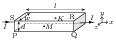# Magnetic Moment of a Current Loop

## Problems from IIT JEE

Problem (IIT JEE 2000):A particle of charge $q$ and mass $m$ moves in a circular orbit of radius $r$ with angular speed $\omega$. The ratio of the magnitude of its magnetic moment to that of its angular momentum depends on

1. $\omega$ and $q$
2. $\omega$, $q$ and $m$
3. $q$ and $m$
4. $\omega$ and $m$

Solution: The angular momentum of the charge of mass $m$ moving in a circular orbit of radius $r$ with angular speed $\omega$ is given by $L=m\omega r^2$. The charge $q$ takes time $T={2\pi}/{\omega}$ to complete one rotation. The average current in the loop and its magnetic moment are given by, \begin{align} i&=\frac{q}{T}=\frac{q\omega}{2\pi}, & \mu&=iA=\frac{q\omega}{2\pi}\;\pi r^2=\frac{1}{2}q\omega r^2. \nonumber \end{align} Thus, ratio of magnetic moment and angular momentum, $\frac{\mu}{L}=\frac{q}{2m}$, depends on $q$ and $m$. The ratio is independent of dynamics parameters i.e., $\omega$ and $r$. This ratio for an electron (charge $e$, mass $m_e$) defines Bohr Magneton, $\mu_B=\frac{h}{2\pi}\frac{\mu}{L}=\frac{eh}{4\pi m_e}$, a physical constant.